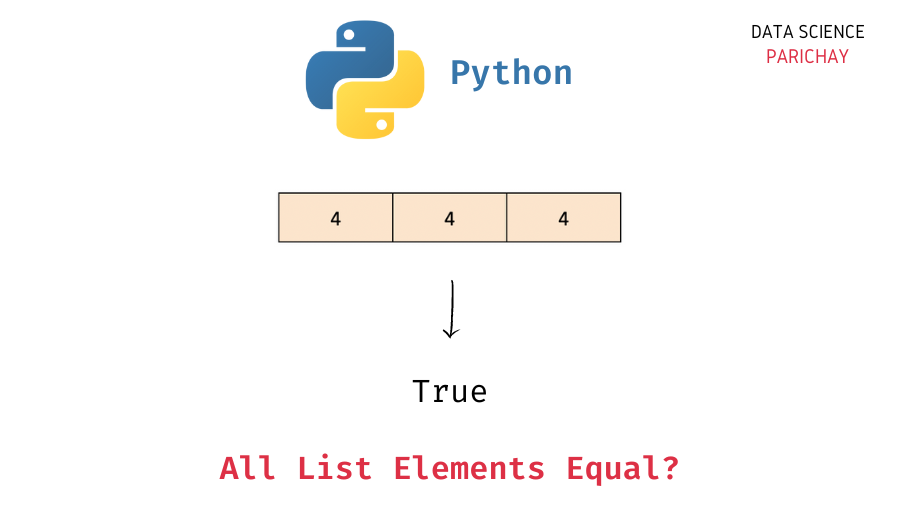# Python – Check If All Elements in a List are Equal

In this tutorial, we will look at how to check if all the elements in a list are equal or not in Python with the help of some examples.

## How to check if all the list items are the same?

You can use the Python built-in `all()` function to check if all the elements in a list are equal or not by checking if each value is the same as the first value in the list.

The `all()` function takes in an iterable as an argument and returns `True` if all the values in the iterable are truthy (represent `True` in a boolean context).

So, to check if all the values in a given list are the same or not, use the `all()` function to check if all the values are equal to the first value in the list. The following is the syntax –

```# check if all the list values are the same
all(val == ls for val in ls)```

It returns `True` if all the values in the list are equal.

Note that there are other methods as well that you can use to check if all list values are the same or not. Some of them are –

• Iterate through the list and keep track of the distinct values you encounter.
• Create a set from list elements and check if its size is one or not.

## Examples

Let’s now look at some examples of using the above methods. First, we will create a few lists that we’ll use to demonstrate the methods.

```# list with all values same
ls1 = [5, 5, 5, 5]
# list with more than one unique value
ls2 = [1, 2, 3, 4, 5, 5]
# empty list
ls3 = []

# display the lists
print(ls1)
print(ls2)
print(ls3)```

Output:

📚 Data Science Programs By Skill Level

Introductory

Intermediate ⭐⭐⭐

🔎 Find Data Science Programs 👨‍💻 111,889 already enrolled

Disclaimer: Data Science Parichay is reader supported. When you purchase a course through a link on this site, we may earn a small commission at no additional cost to you. Earned commissions help support this website and its team of writers.

```[5, 5, 5, 5]
[1, 2, 3, 4, 5, 5]
[]```

Here, we created three lists – `ls1`, `ls2`, and `ls3`. The list `ls1` contains the same value as its elements. The list `ls2` has repeated values but not all values are the same and the list `ls3` is empty (it does not contain any elements).

### Example 1 – Check if all the list elements are equal using `all()`

The idea here is to use the `all()` function to check if each list element is equal to the first list element.

You can use a list comprehension to create a list of boolean values – whether a list element is the same as the first list value and then pass this resulting list as an argument to the `all()` function.

Let’s apply this to the lists `ls1` and `ls2` created above.

```# check if all list values are same
print(all([val == ls1 for val in ls1]))
print(all([val == ls2 for val in ls2]))```

Output:

```True
False```

We get `True` for `ls1` and `False` for `ls2`.

If you apply this method to an empty list, you’ll get `True` as the output.

`all([val == ls3 for val in ls3])`

Output:

`True`

Note that the `all()` takes an iterable as an argument, you can directly use an iterator (without using a list comprehension).

```# check if all list values are same
print(all(val == ls1 for val in ls1))
print(all(val == ls2 for val in ls2))
print(all(val == ls3 for val in ls3))```

Output:

```True
False
True```

We get the same results as above.

### Example 2 – Check if all list elements are equal using a for loop

The idea here is to iterate through the list and keep a count of each unique element we encounter. If the resulting count is 1, we can say that all the values in the list are the same (the list has only one unique value).

You can use another list to keep track of the visited elements.

```def check_if_list_values_same(ls):
visited = []
count = 0
for val in ls:
if val not in visited:
count += 1
visited.append(val)
return count == 1

# check if all list values are same
print(check_if_list_values_same(ls1))
print(check_if_list_values_same(ls2))
print(check_if_list_values_same(ls3))```

Output:

```True
False
False```

We get `True` for `ls1` and `False` for `ls2` and `ls3`. Note that here we get `False` for an empty list.

If you want `True` as the output for an empty list, modify the condition in the return statement to `count <= 1`

### Example 3 – Check if all the list elements are equal using a set

In this method, we create a set from the list elements and check if the size of the set is equal to one. Since a set only holds unique values, if the list has the same elements, the resulting set will only have one value.

```# check if all list values are same
print(len(set(ls1)) == 1)
print(len(set(ls2)) == 1)
print(len(set(ls3)) == 1)```

Output:

```True
False
False```

We get `True` for `ls1` and `False` for `ls2` and `ls3`. (Same as the above method).

If you want `True` as the output for an empty list, check if the set length is less than equal to 1.

## Summary

In this tutorial, we looked at some different methods to check if all the values in a list are the same or not. The following are the different methods covered –

• Use the Python built-in `all()` function to check if each list element is equal to the first list element.
• Iterate through the list elements and track the count of unique values encountered.
• Convert the list to a set and check if its size is equal to one.

You might also be interested in –

•